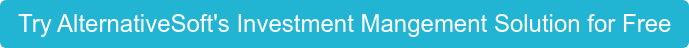640+ Users

###### Best Risk management Software

2021 | 2020 | 2019

# Demystifying the Sharpe Ratio Equation

The Sharpe Ratio is a fundamental tool for assessing the risk-adjusted return of your investments. Dive into this essential metric, demystify its equation, and elevate your understanding of portfolio performance.

## Understanding the Sharpe Ratio

The Sharpe Ratio is more than just a number; it's a key indicator of how efficiently your investments are generating returns relative to the level of risk taken. In this comprehensive guide, we break down the Sharpe Ratio equation into its core components.

## Risk and Reward Balance

At its essence, the Sharpe Ratio quantifies the relationship between:

• The excess return on your investment (the return above a risk-free rate)
• The volatility or standard deviation of those returns (a measure of risk)

## Key Components Unveiled

Our guide will walk you through:

• The Risk-Free Rate: Understand why the risk-free rate is a critical component of the Sharpe Ratio and how it reflects the opportunity cost of investing.

• Portfolio Return: Learn how to calculate the average return on your investment portfolio, considering both gains and losses.

• Volatility Measurement: Demystify the standard deviation, a statistical measure that quantifies the spread of investment returns.

## The Sharpe Ratio in Action

See the Sharpe Ratio in action through practical examples and real-world scenarios. Explore how different investments and portfolio compositions can impact the ratio and influence your investment decisions.

A strong grasp of the Sharpe Ratio empowers you to:

• Assess the risk-return profile of your investments
• Compare different investment options effectively

## Unlock the Power of the Sharpe Ratio Equation

Ready to demystify the Sharpe Ratio equation and elevate your investment acumen? Dive into our guide and gain the knowledge needed to make data-driven investment decisions that align with your financial goals.

## Get Started Today

Don't let the complexities of investment metrics deter you. Understanding the Sharpe Ratio equation is a vital step toward making informed, strategic investment choices. Begin your journey to financial mastery now.